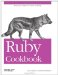# Generating a Sequence of Numbers

Problem

You want to iterate over a (possibly infinite) sequence of numbers the way you can iterate over an array or a range.

Solution

Write a generator function that yields each number in the sequence.

```	def fibonacci(limit = nil)
seed1 = 0
seed2 = 1
while not limit or seed2 <= limit
yield seed2
seed1, seed2 = seed2, seed1 + seed2
end
end

fibonacci(3) { |x| puts x }
# 1
# 1
# 2
# 3

fibonacci(1) { |x| puts x }
# 1
# 1

fibonacci { |x| break if x > 20; puts x }
# 1
# 1
# 2
# 3
# 5
# 8
# 13
```

Discussion

A generator for a sequence of numbers works just like one that iterates over an array or other data structure. The main difference is that iterations over a data structure usually have a natural stopping point, whereas most common number sequences are infinite.

One strategy is to implement a method called each that yields the entire sequence. This works especially well if the sequence is finite. If not, it's the responsibility of the code block that consumes the sequence to stop the iteration with the break keyword.

Range#each is an example of an iterator over a finite sequence, while Prime#each enumerates the infinite set of prime numbers. Range#each is implemented in C, but here's a (much slower) pure Ruby implementation for study. This code uses self.begin and self.end to call Range#begin and Range#end, because begin and end are reserved words in Ruby.

```	class Range
def each_slow
x = self.begin
while x <= self.end
yield x
x = x.succ
end
end
end

(1..3).each_slow {|x| puts x}
# 1
# 2
# 3
```

The other kind of sequence generator iterates over a finite portion of an infinite sequence. These are methods like Fixnum#upto and Fixnum#step: they take a start and/ or an end point as input, and generate a finite sequence within those boundaries.

```	class Fixnum
def double_upto(stop)
x = self
until x > stop
yield x
x = x * 2
end
end
end
10.double_upto(50) { |x| puts x }
# 10
# 20
# 40
```

Most sequences move monotonically up or down, but it doesn't have to be that way:

```	def oscillator
x = 1
while true
yield x
x *= -2
end
end
oscillator { |x| puts x; break if x.abs > 50; }
# 1
# -2
# 4
# -8
# 16
# -32
# 64
```

Though integer sequences are the most common, any type of number can be used in a sequence. For instance, Float#step works just like Integer#step:

```	1.5.step(2.0, 0.25) { |x| puts x }
# => 1.5
# => 1.75
# => 2.0
```

Float objects don't have the resolution to represent every real number. Very small differences between numbers are lost. This means that some Float sequences you might think would go on forever will eventually end:

```	def zeno(start, stop)
distance = stop - start
travelled = start
while travelled < stop and distance > 0
yield travelled
distance = distance / 2.0
travelled += distance
end
end

steps = 0
zeno(0, 1) { steps += 1 }
steps # => 54
```

• Recipe 1.16, " Generating a Succession of Strings"
• Recipe 2.16, "Generating Prime Numbers," shows optimizations for generating a very well-studied number sequence
• Recipe 4.1, "Iterating Over an Array"
• Chapter 7 has more on this kind of generator methodRuby Cookbook (Cookbooks (OReilly))
ISBN: 0596523696
EAN: 2147483647
Year: N/A
Pages: 399

Similar book on Amazon